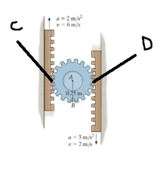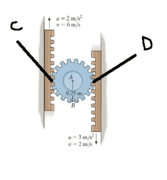# Centripetal acceleration help?

tandennis0703

## Homework Statement

Hi, I was wondering what is the difference between the equations an=w^2 x R vs an=V^2 / R? I thought both of these equations were the same? What is the normal acceleration at C and D?## Homework Equations

an=w^2 x R vs an=V^2 / R?

## The Attempt at a Solution

[/B]
If I use the equation w^2 R for the normal component

At point C I get (16)^2 x (0.25) = 64ms-2
At point D I get (16)^2 x (0.25) = 64ms-2

However, if I use the equation v^2 / R for the normal component

At point C I get (6)^2 / (0.25) = 144ms-2
At point D I get (2)^2 / (0.25) = 16ms-2

Why is this so?

Gold Member

## Homework Statement

Hi, I was wondering what is the difference between the equations an=w^2 x R vs an=V^2 / R? I thought both of these equations were the same? What is the normal acceleration at C and D?## Homework Equations

an=w^2 x R vs an=V^2 / R?

## The Attempt at a Solution

[/B]
If I use the equation w^2 R for the normal component

At point C I get (16)^2 x (0.25) = 64ms-2
At point D I get (16)^2 x (0.25) = 64ms-2

However, if I use the equation v^2 / R for the normal component

At point C I get (6)^2 / (0.25) = 144ms-2
At point D I get (2)^2 / (0.25) = 16ms-2

Why is this so?

How are you defining ##\omega##? Check your math.

tandennis0703
How are you defining ##\omega##? Check your math.

My maths is fine. I have the answer which is w=16.

To get w you need to equate both VD and VC by using the radius r as the IC point.

Vd=wx ; 6=wx
Vc=w(0.5-x) ; 2=w(0.5-x)

Therefore by substitution, you get the angular velocity equals to 16 ms-1. I hope this helps you understand how to get the angular velocity. If you need any help feel free to contact me.

Mentor
The velocity used in v2/r should be the tangential velocity with respect to the center of rotation. Here the center of rotation is at the center of the circular gear, which is in motion (linear motion upwards in the figure). So for example, on the left had side the motion of the contact point C in the "lab frame" is 6 m/s upwards. But the center of the gear is moving upwards with some velocity v. So the tangential velocity with respect to the center of rotation will be.....?

Hint: Find the speed of the center of mass of the gear.

Gold Member
My maths is fine. I have the answer which is w=16.

To get w you need to equate both VD and VC by using the radius r as the IC point.

Vd=wx ; 6=wx
Vc=w(0.5-x) ; 2=w(0.5-x)

Therefore by substitution, you get the angular velocity equals to 16 ms-1. I hope this helps you understand how to get the angular velocity. If you need any help feel free to contact me.

Or you could just equate the formulas you're using ##\frac{v^2}{r}=\omega^2r## solve for ##\omega## and see there is a problem.

Last edited by a moderator:
tandennis0703
The velocity used in v2/r should be the tangential velocity with respect to the center of rotation. Here the center of rotation is at the center of the circular gear, which is in motion (linear motion upwards in the figure). So for example, on the left had side the motion of the contact point C in the "lab frame" is 6 m/s upwards. But the center of the gear is moving upwards with some velocity v. So the tangential velocity with respect to the center of rotation will be.....?

Hint: Find the speed of the center of mass of the gear.

Oh no I did not see the question correctly. Both the velocity at C and D of the WHEEL is the same. It is the side belt thats moving at a velocity.

tandennis0703
Or you could just equate the formulas you're using ##\frac{v^2}{r}=\omega^2r## solve for ##\omega## and see there is a problem.

The velocities at C and D are the same. The conveyor is the one that is moving at 6 and 2 ms-1 respectively. Therefore I am not able to find the normal acceleration by using the velocities of the conveyor belt. The velocities at B,C, and D of the wheel are constant, so I can use either velocities in the equation v^2/R. Or even easier, just use the angular velocity an=w^2 R. Thanks for your help!